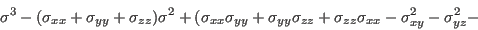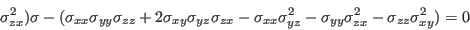## Principal Stresses

Entity names: PS1, PS2, PS3, worstPS

The principal stresses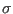are named PS1, PS2, PS3. From the three principal stressesthe absolute maximum value will be calculated and named worstPS. For example if a node has the three values 100, 0 and -110 MPa then -110 MPa would be shown. The three principal stresses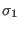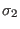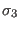are derived from the following equation: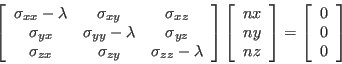They are given by the three roots of the equation (stress tensor is symmetric: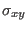=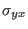etc.):